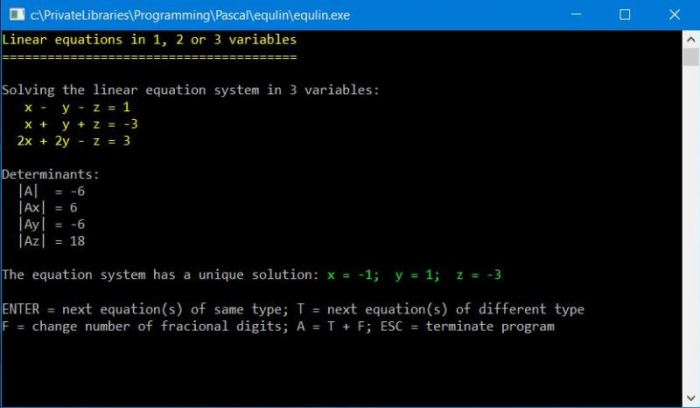# Computing: Free Pascal Programming

 Programming Home Home Contact

## Linear equation systems in 1, 2 or 3 variables (equlin).

Description: The program solves linear equations in one variable and linear equation systems in two or three variables (2-by-2 and 3-by-3 systems). All the user has to do, is choosing the equation type (number of variables) and entering the coefficients. The program calculates the unique solution for x (1 variable), x and y (2 variables) resp. x, y and z (3 variables), if it exists. Otherwise, it indicates if the equation system has an infinity or no solution at all.
Equations in 1 variable are considered to have the format: M1(A1x + D1) = M2(A2x + D2). This allows to resolve exercises that use coefficient multipliers, without having to do the multiplication manually. Equations of 2 variables systems and 3 variables systems use the classic format: Ax + By = D resp. Ax + By + Cz = D; their solution is done by using Cramer's determinant method
After the solution has been displayed, hit ENTER to continue with next equation of same type, hit 'T' to change the equation type, hit ESC to terminate the program.

Change log:
Version 1.0 (April 2018): Original program.
Version 1.1 (July 2019):  Display of the determinant values (for the 2- and 3-variables equations).

Free Pascal features: Number input with numeric-checking and default value; formatted output of real numbers; decision making on keyboard key pressed; "extending" Free Pascal mathematics by writing own functions.

Screenshot: